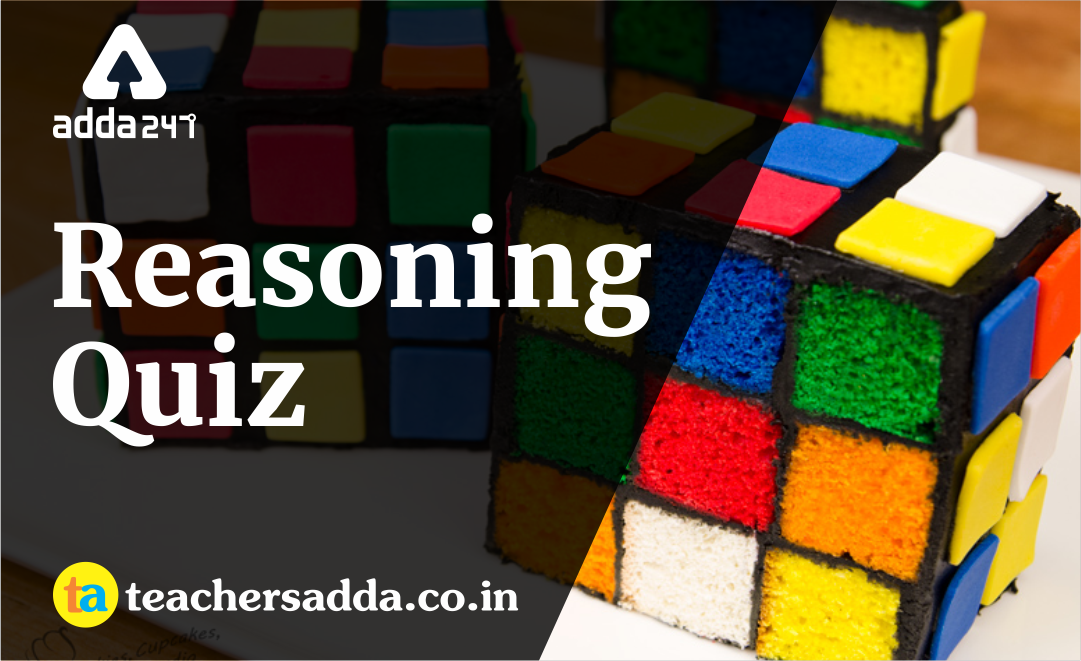The reasoning is the most scoring section in any competitive exam. It also develops or nourishes our critical thinking skills as compared to other subjects. In DSSSB, KVS Exam as a student to score well in this part, you have to start your preparation now because there is negative marking as well. The more you have prepared, the better you score.

Q1. If ‘POST’ is coded as ‘KLHG’, how is ‘NURS’ coded as?
(a) MFJH
(b) MGJH
(c) MFIH
(d) MFIG

Q2. If GARMENT is written as 202691422137, how is INDULGE written in that code?
(a) 9144211275
(b) 914211275
(c) 1813326152022
(d) 1813236152022

Directions (3-7): In each of the following questions, select the missing number from the given responses.

Q3.
? 3
9 5
(a) 14
(b) 15
(c) 12
(d) 13

Q4.
R Q L
S P M
T ? N
(a) O
(b) W
(c) R
(d) V

Q5.
7 6 15
10 ? 12
35 12 90
(a) 9
(b) 25
(c) 4
(d) 11

Q6. If ‘+’ means ‘÷’; ‘÷’ means ‘–’; ‘–’ means ‘×’; ‘×’ means ‘+’, then
8 + 2 ÷ 3 – 4 × 6 =?
(a) –12
(b) –2
(c) –10
(d) –15

Q7. Choose the appropriate combination of signs to solve.
16*8*1*8
(a) = – ÷
(b) – ÷ =
(c) ÷ – =
(d) ÷ = –

Q8. The percentage of Laptop users are increasing year after year in India. Find out the percentage of Laptop users for the year 2011 from the following information.(a) 62
(b) 66
(c) 60
(d) 64

Q9. Raghu starts from his house in his car and travels 8 km towards the North, then 6 km towards East then 10 km towards his right, 4 km towards his left, 10 km towards North and finally 4 km towards his right. In which direction is he now with reference to the starting point?
(a) South
(b) North East
(c) South East
(d) North

Q10. Four students ABCD are sitting one each at the four corners of a square all facing the centre of the square. The student E sitting at the centre is facing only C and the student A is sitting facing the back of E. If D is sitting on the right of E, where B will be sitting to E?
(a) B is sitting on the left of E
(b) B is to the back of E
(c) A is facing B and E
(d) B is on the right of E

Solutions

S1. Ans. (c)
Pairs of opposite letters.
Similarly,

S2. Ans. (d)
Sol. G ⇒ 27 – 7 = 20
A ⇒ 27 – 1 = 26
R ⇒ 27 – 18 = 9
M ⇒ 27 – 13 = 14
E ⇒ 27 – 5 = 22
N ⇒ 27 – 14 = 13
T ⇒ 27 – 20 = 7
Similarly,
I ⇒ 27 – 9 = 18
N ⇒ 27 – 14 = 13
D ⇒ 27 – 4 = 23
U ⇒ 27 – 21 = 6
L ⇒ 27 – 12 = 15
G ⇒ 27 – 7 = 20
E ⇒ 27 – 5 = 22

S3. Ans. (b)
Sol. 3 + 2 = 5
5 + 4 = 9
9 + 6 =

S4. Ans. (a)
Sol. Column wise

S5. Ans. (c)
Sol. Column wise
(7×10)/2=35
(6×?)/2=12
⇒?=12/3=
(15×12)/2=90

S6. Ans. (b)
Sol.
8 + 2 ÷ 3 – 4 × 6 =?
⇒ ? = 8 ÷ 2 – 3 × 4 + 6
⇒ ? = 4 – 12 + 6 = – 2

S7. Ans. (b)
Sol. 16 – 8 ÷ 1 = 8
⇒ 16 – 8 = 8

S8. Ans. (d)
Sol. 2006 to 2007
4 + 4 = 8
2007 to 2008
8 + 8 = 16
2008 to 2009
16 + 12 = 28
2009 to 2010
28 + 16 = 44
2010 to 2011
44 + 20 =

S9. Ans. (b)
Sol.

S10. Ans. (a)
Sol.
B is sitting on the left of E.

Get FREE Study Material For UGC NET

You may also like to read :